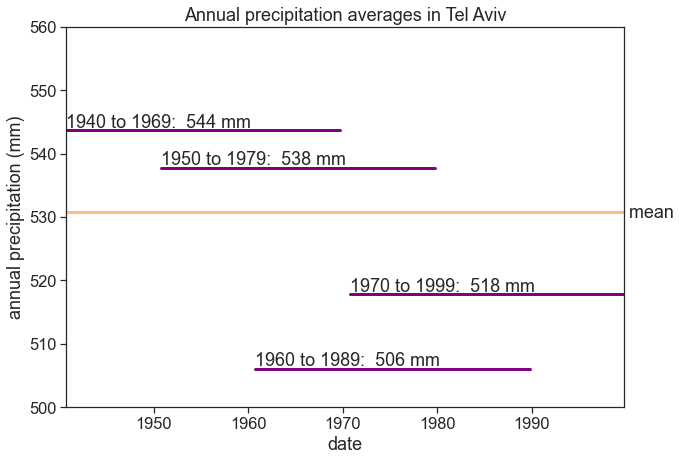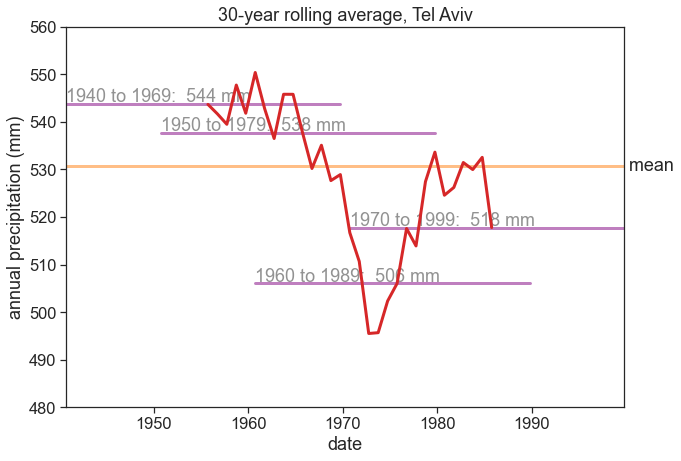import matplotlib.pyplot as plt
import matplotlib
import numpy as np
import pandas as pd
from pandas.plotting import register_matplotlib_converters
register_matplotlib_converters()  # datetime converter for a matplotlib
from calendar import month_abbr
import seaborn as sns
sns.set(style="ticks", font_scale=1.5)
import urllib.request

def download_data(station_name, station_code):
url_daily = 'https://www.ncei.noaa.gov/data/global-historical-climatology-network-daily/access/'
url_monthly = 'https://www.ncei.noaa.gov/data/gsom/access/'
# download daily data
urllib.request.urlretrieve(url_daily + station_code + '.csv',
station_name + '_daily.csv')
# download monthly data
urllib.request.urlretrieve(url_monthly + station_code + '.csv',
station_name + '_monthly.csv')

df = pd.read_csv("TEL AVIV READING_monthly.csv", sep=",")
# make 'DATE' the dataframe index
df['DATE'] = pd.to_datetime(df['DATE'])
df = df.set_index('DATE')
df

STATION LATITUDE LONGITUDE ELEVATION NAME CDSD CDSD_ATTRIBUTES CLDD CLDD_ATTRIBUTES DP01 ... HTDD HTDD_ATTRIBUTES PRCP PRCP_ATTRIBUTES TAVG TAVG_ATTRIBUTES TMAX TMAX_ATTRIBUTES TMIN TMIN_ATTRIBUTES
DATE
1939-11-01 IS000002011 32.1 34.7831 3.0 TEL AVIV READING, IS NaN NaN NaN NaN 7.0 ... NaN NaN 106.5 ,,,E NaN NaN NaN NaN NaN NaN
1939-12-01 IS000002011 32.1 34.7831 3.0 TEL AVIV READING, IS NaN NaN NaN NaN 7.0 ... NaN NaN 100.1 ,,,E NaN NaN NaN NaN NaN NaN
1940-01-01 IS000002011 32.1 34.7831 3.0 TEL AVIV READING, IS NaN NaN NaN NaN 11.0 ... NaN NaN 181.5 ,,,E NaN NaN NaN NaN NaN NaN
1940-02-01 IS000002011 32.1 34.7831 3.0 TEL AVIV READING, IS NaN NaN NaN NaN 6.0 ... NaN NaN 57.7 ,,,E NaN NaN NaN NaN NaN NaN
1940-03-01 IS000002011 32.1 34.7831 3.0 TEL AVIV READING, IS NaN NaN NaN NaN 7.0 ... NaN NaN 27.2 ,,,E NaN NaN NaN NaN NaN NaN
... ... ... ... ... ... ... ... ... ... ... ... ... ... ... ... ... ... ... ... ... ...
1999-05-01 IS000002011 32.1 34.7831 3.0 TEL AVIV READING, IS 165.9 NaN 125.0 ,I NaN ... 0.0 ,I NaN NaN 22.37 ,I 26.15 ,,,I 18.58 ,,,I
1999-06-01 IS000002011 32.1 34.7831 3.0 TEL AVIV READING, IS 367.1 NaN 201.2 1,I NaN ... 0.0 1,I NaN NaN 25.29 1,I 28.93 1,,,I 21.65 ,,,I
1999-07-01 IS000002011 32.1 34.7831 3.0 TEL AVIV READING, IS 612.0 NaN 244.9 4,I NaN ... 0.0 4,I NaN NaN 27.44 4,I 31.09 4,,,I 23.80 ,,,I
1999-08-01 IS000002011 32.1 34.7831 3.0 TEL AVIV READING, IS 921.4 NaN 309.4 ,I NaN ... 0.0 ,I NaN NaN 28.31 ,I 31.54 ,,,I 25.09 ,,,I
1999-11-01 IS000002011 32.1 34.7831 3.0 TEL AVIV READING, IS NaN NaN 62.1 ,I NaN ... 13.3 ,I NaN NaN 19.96 ,I 24.41 ,,,I 15.51 ,,,I

719 rows × 43 columns

fig, (ax1, ax2) = plt.subplots(2, 1, figsize=(10,7))

# plot precipitation
ax1.fill_between(df.index, df['PRCP'], 0, color='tab:blue')
df_1990_1992 = df.loc['1990-07-01':'1992-07-01']
ax2.bar(df_1990_1992.index, df_1990_1992['PRCP'], width=30)

# adjust labels, ticks, title, etc
ax1.set_title("Monthly precipitation in Tel Aviv")
ax2.tick_params(axis='x', rotation=45)
ax2.set_xlabel("date")

# dirty trick to get common y label between the two panels:
# make a large invisible axes, give it a ylabel
ax0 = fig.add_subplot(111, frame_on=False)
ax0.tick_params(labelcolor="none", bottom=False, left=False)
ax0.set_ylabel("monthly precipitation (mm)", labelpad=20)

# write yearly rainfall
rain_1990_1991 = df.loc['1990-07-01':'1991-07-01','PRCP'].sum()
rain_1991_1992 = df.loc['1991-07-01':'1992-07-01','PRCP'].sum()
ax2.text('1991-01-01', 300, "{:.0f} mm".format(rain_1990_1991))
ax2.text('1992-01-01', 300, "{:.0f} mm".format(rain_1991_1992))

# save figure
plt.savefig("monthly_tel_aviv_1940-1999.png")## hydrological year

A time period of 12 months for which precipitation totals are measured. The water year is designated by the calendar year in which it ends.
Let's define the hydrological year for Tel Aviv from 1 October to 30 September.

# read more about resampling options
# https://pandas.pydata.org/pandas-docs/version/0.12.0/timeseries.html#offset-aliases
# also, annual resampling can be anchored to the end of specific months:
# https://pandas.pydata.org/pandas-docs/version/0.12.0/timeseries.html#anchored-offsets
df_year_all = df['PRCP'].resample('A-SEP').sum().to_frame()  # annual frequency, anchored end of September
df_year_all.columns = ['rain (mm)'] # rename 'PRCP' column to 'rain (mm)'
df_year_all

rain (mm)
DATE
1940-09-30 474.9
1941-09-30 447.8
1942-09-30 372.9
1943-09-30 618.2
1944-09-30 440.5
... ...
1996-09-30 488.2
1997-09-30 619.1
1998-09-30 489.6
1999-09-30 226.5
2000-09-30 0.0

61 rows × 1 columns

# the last year is the sum of only on month (November), let's take it out
df_year = df_year_all.iloc[:-1]  # exclude last row
df_year.tail()  # show "tail" of the dataframe to see that year 2000 was excluded

rain (mm)
DATE
1995-09-30 804.7
1996-09-30 488.2
1997-09-30 619.1
1998-09-30 489.6
1999-09-30 226.5
fig, ax = plt.subplots(figsize=(10,7))

# plot YEARLY precipitation
ax.bar(df_year.index, df_year['rain (mm)'],
width=365, align='edge', color="tab:blue")

# plot mean
rain_mean = df_year['rain (mm)'].mean()
ax.plot(df_year*0 + rain_mean, linewidth=3, color="tab:orange")

# adjust labels, ticks, title, etc
ax.set_title("Annual precipitation in Tel Aviv, 1940–1999")
ax.set_xlabel("date")
ax.set_ylabel("annual precipitation (mm)")
ax.set_xlim([df_year.index, df_year.index[-1]])

# write mean on the right
ax.text(df_year.index[-1], rain_mean, " mean\n {:.0f} mm".format(rain_mean),
horizontalalignment="left", verticalalignment="center")

# save figure
plt.savefig("annual_tel_aviv_with_mean.png")fig, ax = plt.subplots(figsize=(10,7))

# calculate mean and standard deviation
rain_mean = df_year['rain (mm)'].mean()
rain_std = df_year['rain (mm)'].std()

# plot histogram
b = np.arange(0, 1101, 100)  # bins from 0 to 55, width = 5
ax.hist(df_year, bins=b)

# plot vertical lines with mean, std, etc
ylim = np.array(ax.get_ylim())
ylim = ylim*1.1
ax.plot([rain_mean]*2, ylim, linewidth=3, color="tab:orange")
ax.plot([rain_mean+rain_std]*2, ylim, linewidth=3, linestyle="--", color="tab:olive")
ax.plot([rain_mean-rain_std]*2, ylim, linewidth=3, linestyle="--", color="tab:olive")
ax.set_ylim(ylim)

# write mean, std, etc
ax.text(rain_mean, ylim*0.99, "mean",
horizontalalignment="center",
verticalalignment="top",
bbox=dict(facecolor='white', edgecolor='none', pad=0.0))
ax.text(rain_mean+rain_std, ylim*0.99, "mean+std",
horizontalalignment="center",
verticalalignment="top",
bbox=dict(facecolor='white', edgecolor='none', pad=0.0))
ax.text(rain_mean-rain_std, ylim*0.99, "mean-std",
horizontalalignment="center",
verticalalignment="top",
bbox=dict(facecolor='white', edgecolor='none', pad=0.0))

# adjust labels, ticks, title, limits, etc
ax.set_title("Histogram of annual precipitation in Tel Aviv, 1940–1999")
ax.set_xlabel("annual rainfall (mm)")
ax.set_ylabel("number of years")

# save figure
plt.savefig("histogram_tel_aviv_with_mean_and_std.png")## coefficient of variation

$\langle{P}\rangle=$ average precipitation
$\sigma=$ standard deviation

$$CV = \frac{\sigma}{\langle{P}\rangle}$$

Assuming that the inter-annual distribution is a gaussian: 67% of the time, rainfall will vary +/- 30% from its long term average in Tel Aviv.

CV = rain_std / rain_mean
print(f"CV = {CV:.2f}")
# rain_mean

CV = 0.30

fig, ax = plt.subplots(figsize=(10,7))

# windows of length 30 years
windows = [[1940,1969], [1970,1999]]
for window in windows:
start_date = f"{window:d}-09-30"
end_date = f"{window:d}-09-30"
window_mean = df_year['rain (mm)'][start_date:end_date].mean()
ax.plot(df_year[start_date:end_date]*0+window_mean, color="purple", linewidth=3)
ax.text(start_date, window_mean+0.5, f"{window} to {window}:  {window_mean:.0f} mm")

# plot mean
rain_mean = df_year['rain (mm)'].mean()
ax.plot(df_year*0 + rain_mean, linewidth=3, color="tab:orange", alpha=0.5)
ax.text(df_year.index[-1], rain_mean, " mean".format(rain_mean),
horizontalalignment="left", verticalalignment="center")

# adjust labels, ticks, title, limits, etc
ax.set_title("Annual precipitation averages in Tel Aviv, 1940–1999")
ax.set_xlabel("date")
ax.set_ylabel("annual precipitation (mm)")
ax.set_xlim([df_year.index, df_year.index[-1]])
ax.set_ylim([500, 560])

# save figure
plt.savefig("mean_tel_aviv_2_windows.png")fig, ax = plt.subplots(figsize=(10,7))

# windows of length 30 years
windows = [[x,x+29] for x in [1940,1950,1960,1970]]
for window in windows:
start_date = f"{window:d}-09-30"
end_date = f"{window:d}-09-30"
window_mean = df_year['rain (mm)'][start_date:end_date].mean()
ax.plot(df_year[start_date:end_date]*0+window_mean, color="purple", linewidth=3)
ax.text(start_date, window_mean+0.5, f"{window} to {window}:  {window_mean:.0f} mm")

# plot mean
ax.plot(df_year*0 + rain_mean, linewidth=3, color="tab:orange", alpha=0.5)
ax.text(df_year.index[-1], rain_mean, " mean".format(rain_mean),
horizontalalignment="left", verticalalignment="center")

# adjust labels, ticks, title, limits, etc
ax.set_title("Annual precipitation averages in Tel Aviv")
ax.set_xlabel("date")
ax.set_ylabel("annual precipitation (mm)")
ax.set_xlim([df_year.index, df_year.index[-1]])
ax.set_ylim([500, 560])

# save figure
plt.savefig("mean_tel_aviv_4_windows.png")import altair as alt
from vega_datasets import data

# Altair only recognizes column data; it ignores index values. You can plot the index data by first resetting the index
source = df_year.reset_index()
brush = alt.selection(type='interval', encodings=['x'])

# T: temporal, a time or date value
# Q: quantitative, a continuous real-valued quantity
# https://altair-viz.github.io/user_guide/encoding.html#encoding-data-types
bars = alt.Chart().mark_bar().encode(
x=alt.X('DATE:T', axis=alt.Axis(title='date')),
y=alt.Y('rain (mm):Q',  axis=alt.Axis(title='annual precipitation (mm) and average')),
opacity=alt.condition(brush, alt.OpacityValue(1), alt.OpacityValue(0.2)),
).add_selection(
brush
).properties(
title='Select year range and drag for rolling average of annual precipitation in Tel Aviv'
).properties(
width=600,
height=400
)

line = alt.Chart().mark_rule(color='orange').encode(
y='mean(rain (mm)):Q',
size=alt.SizeValue(3)
).transform_filter(
brush
)

alt.layer(bars, line, data=source)

fig, ax = plt.subplots(figsize=(10,7))

rolling_mean = df_year.rolling(30, center=True).mean()
ax.plot(rolling_mean, linewidth=3, color="tab:red", zorder=5)

ax.set_title("30-year rolling average, Tel Aviv")
ax.set_xlabel("date")
ax.set_ylabel("annual precipitation (mm)")

# windows of length 30 years
windows = [[x,x+29] for x in [1940,1950,1960,1970]]
for window in windows:
start_date = f"{window:d}-09-30"
end_date = f"{window:d}-09-30"
window_mean = df_year['rain (mm)'][start_date:end_date].mean()
ax.plot(df_year[start_date:end_date]*0+window_mean, color="purple", linewidth=3, alpha=0.5)
ax.text(start_date, window_mean+0.5, f"{window} to {window}:  {window_mean:.0f} mm", alpha=0.5)
ax.set_ylim([480, 560])

# plot mean
ax.plot(df_year*0 + rain_mean, linewidth=3, color="tab:orange", alpha=0.5)
ax.text(df_year.index[-1], rain_mean, " mean".format(rain_mean),
horizontalalignment="left", verticalalignment="center")
ax.set_xlim([df_year.index, df_year.index[-1]])

# save figure
plt.savefig("rolling_average_tel_aviv.png")rolling_mean

rain (mm)
DATE
1940-09-30 NaN
1941-09-30 NaN
1942-09-30 NaN
1943-09-30 NaN
1944-09-30 NaN
1945-09-30 NaN
1946-09-30 NaN
1947-09-30 NaN
1948-09-30 NaN
1949-09-30 NaN
1950-09-30 NaN
1951-09-30 NaN
1952-09-30 NaN
1953-09-30 NaN
1954-09-30 NaN
1955-09-30 543.683333
1956-09-30 541.690000
1957-09-30 539.496667
1958-09-30 547.746667
1959-09-30 541.823333
1960-09-30 550.390000
1961-09-30 542.760000
1962-09-30 536.510000
1963-09-30 545.810000
1964-09-30 545.803333
1965-09-30 537.756667
1966-09-30 530.206667
1967-09-30 535.093333
1968-09-30 527.663333
1969-09-30 528.926667
1970-09-30 516.710000
1971-09-30 510.670000
1972-09-30 495.516667
1973-09-30 495.690000
1974-09-30 502.350000
1975-09-30 506.033333
1976-09-30 517.593333
1977-09-30 513.926667
1978-09-30 527.450000
1979-09-30 533.643333
1980-09-30 524.583333
1981-09-30 526.223333
1982-09-30 531.463333
1983-09-30 529.980000
1984-09-30 532.553333
1985-09-30 517.790000
1986-09-30 NaN
1987-09-30 NaN
1988-09-30 NaN
1989-09-30 NaN
1990-09-30 NaN
1991-09-30 NaN
1992-09-30 NaN
1993-09-30 NaN
1994-09-30 NaN
1995-09-30 NaN
1996-09-30 NaN
1997-09-30 NaN
1998-09-30 NaN
1999-09-30 NaN